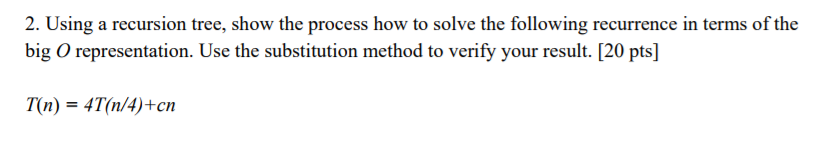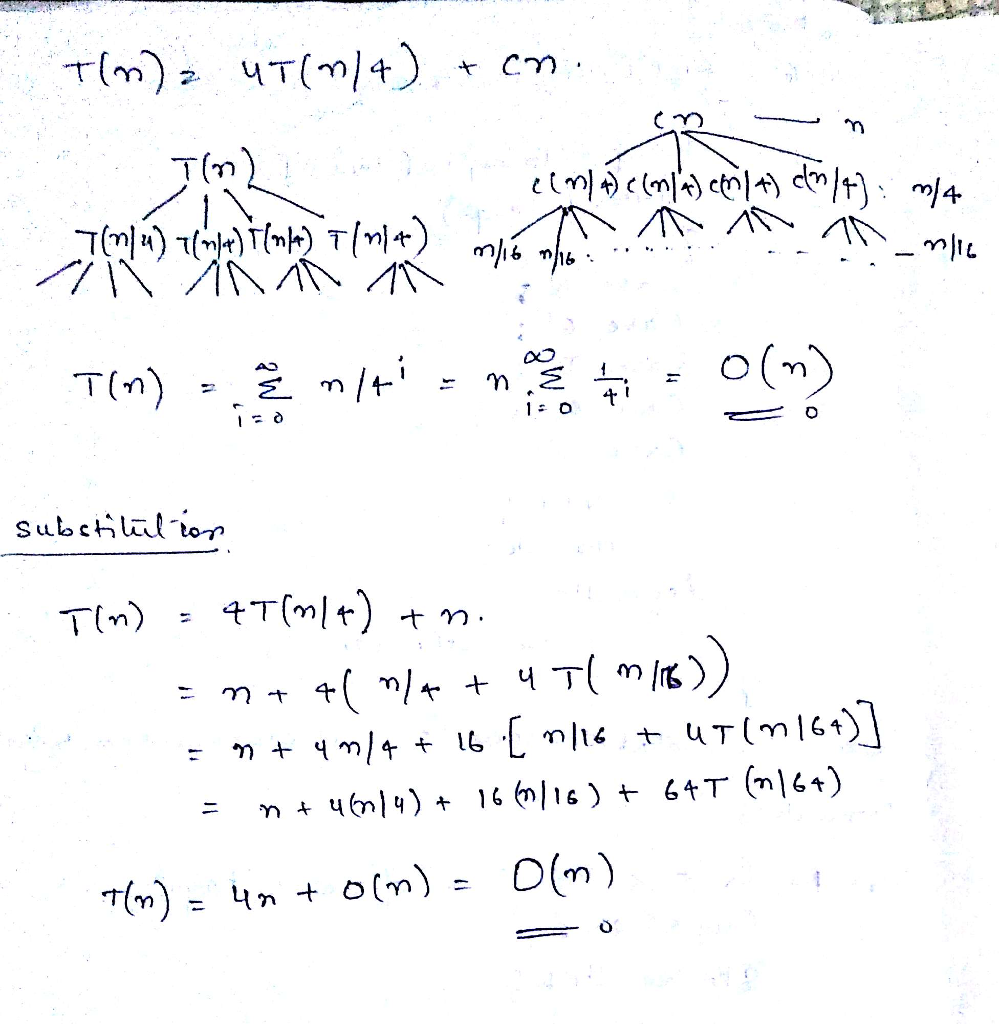# Question & Answer: Using a recursion tree, show the process how to solve the following recurrence in terms of t…..Using a recursion tree, show the process how to solve the following recurrence in terms of the big O representation. Use the substitution method to verify your result. T(n) = 4T(n/4)+cn